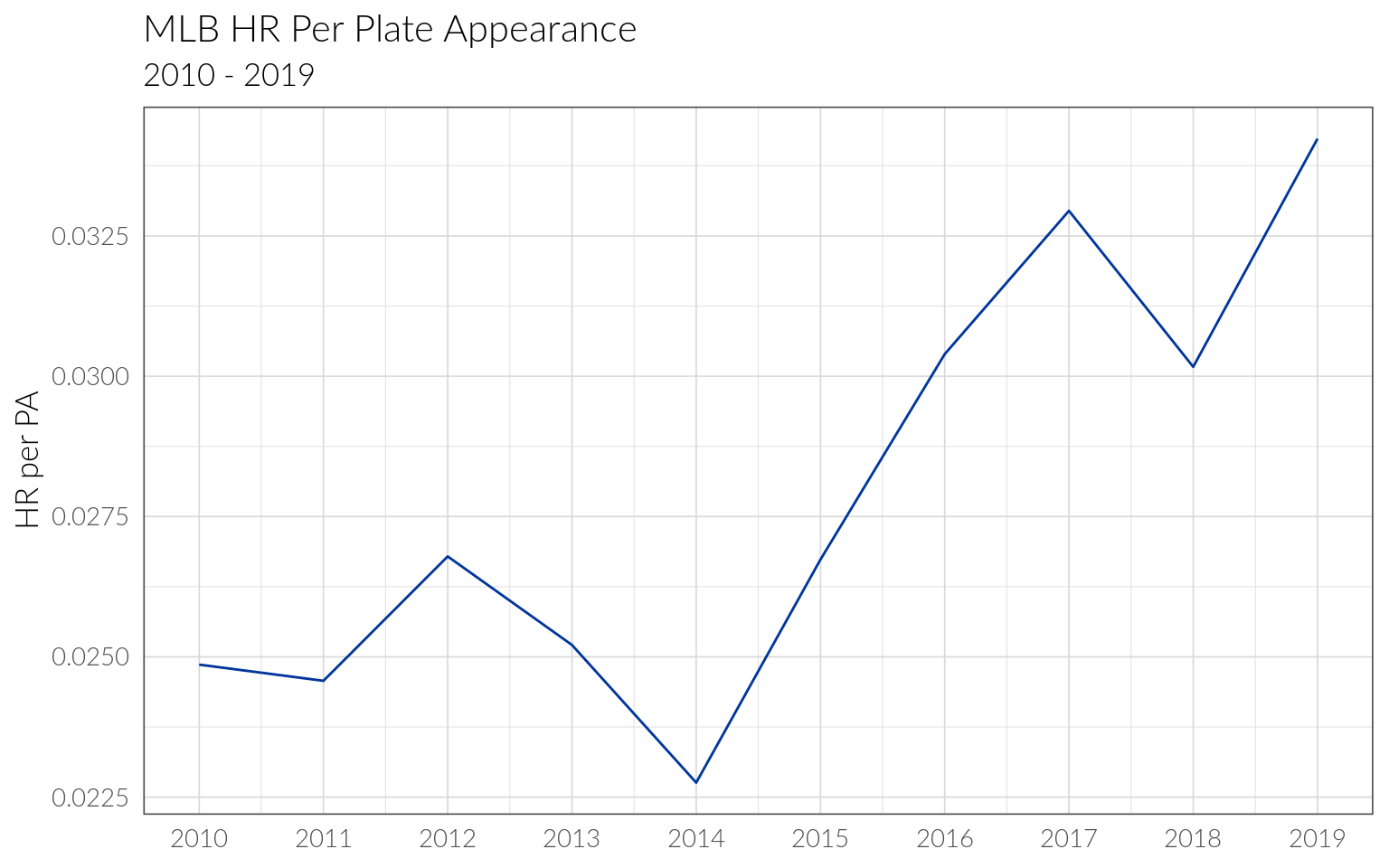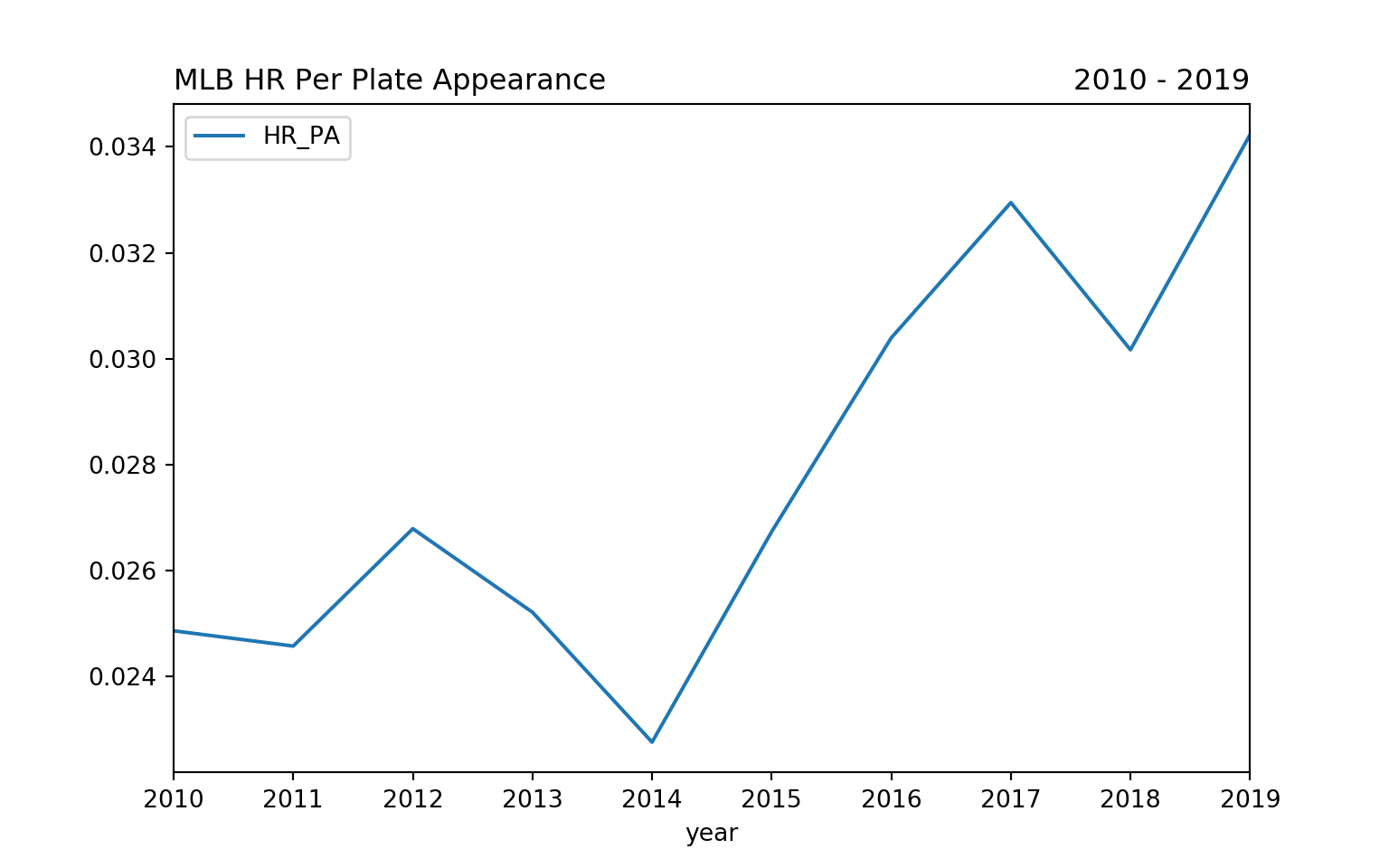# mdgbeck.com

## Michael Groesbeck’s website

RStudio version 1.2 comes with built in support for python using the reticulate package. This is a quick write up of my set up process in Ubuntu, as well as a simple visualization of MLB batting data using R and Python in the same notebook.

## Setting up the virtual environment

I have been using Pipenv for managing my python packages and virtual environments, and the reticulate documentation includes instructions on how to use previously save environments within RStudio. The reticulate::virtualenv_list() lists all virtual environments saved in a specific location. By default, it looks in ~/.virtualenvs, but this behavior can be changed by defining the system environment variable WORKON_HOME as any other file location. In Ubuntu, I found the easiest way to do this was adding the variable to my /etc/environment file, then logging out and back in. Once I did this, running the reticulate::virtualenv_list() command displayed my saved environments. I then specified which to use with the function reticulate::use_virtualenv(). After this, I could now import the various python packages installed in that environment. And if I need to install additional packages, I can use the Terminal pane and quickly install packages with pipenv.

## Using Python

With the environment setup, we can now use the pybaseball package to pull 10 seasons of batting data from baseball-reference.com.

import pandas as pd
from pybaseball import batting_stats_bref

def get_batting(year):
df = batting_stats_bref(year)
df['year'] = year
return df

df = pd.concat([get_batting(year) for year in range(2010, 2020)], ignore_index=True)

We can then reference the data in R with the following code. Note, the library reticulate must be loaded or the py object will not be recognized.

library(reticulate)
batting <- py\$df

The home run rate this season is higher than it has ever been in MLB history. We can create a visualization of the HR rate per plate appearance over the last 10 seasons.

hr_rate <- batting %>%
group_by(year) %>%
summarise(
HR = sum(HR),
PA = sum(PA),
HR_PA = HR / PA
)

ggplot(hr_rate, aes(year, HR_PA)) +
geom_line(color = "#00369C") +
labs(title = "MLB HR Per Plate Appearance",
subtitle = "2010 - 2019",
y = "HR per PA") +
scale_x_continuous(breaks = 2010:2019) +
theme(axis.title.x = element_blank())We can then call the summarized data in python to plot the same thing using matplotlib.

import matplotlib.pyplot as plt
df = r.hr_rate

df.plot(kind="line",x="year",y="HR_PA")
plt.title("MLB HR Per Plate Appearance", loc="left")
plt.title("2010 - 2019", loc="right")